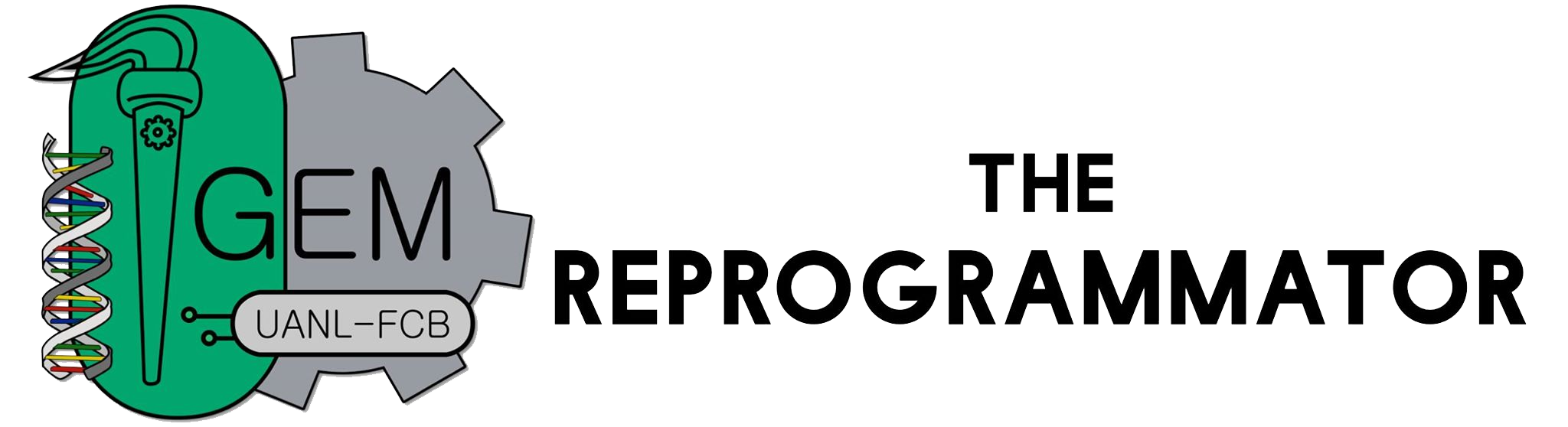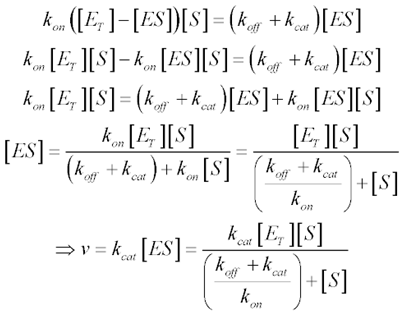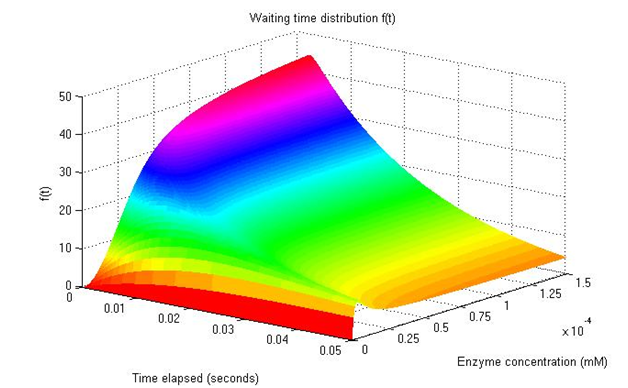# Team:UANL Mty-Mexico/MathModel/talenMath Model
 Talen Activity Model
 Math modeling is a very useful tool, a way for us to accurately describe a system. In our project, the objective is to demonstrate ZFN/TALEN mediated degradation of plasmids within Escherichia coli. Specifically, it would be interesting to see how the protein concentration affects the rate at which the plasmids are degraded. In theory, one only needs a single protein (or two, in case of dimers) to interact with their specific DNA binding site and cut the strands. However, our plasmid and proteins are not alone within a cell. We now present a way to describe how protein concentration has an impact on the speed at which the target plasmid is degraded. ZFN and TALEN both have an enzymatic component, the nuclease. Enzymatic activity is commonly described using the Michaelis-Menten kinetics:Which take into account enzyme and substrate concentrations, and also the enzyme’s on-off mechanism that limits the reaction rate. While this method of describing normal enzymatic reactions, it is not really suitable for our specific needs. Given that only a few proteins will be produced before the plasmid is cut, it does not make much sense to use concentrations, as the value would be too low. In the same way, there would only be a limited number of plasmids to be cut. One way to more accurately describe our cell system is to modify the Michaelis-Mentenequations from using enzyme and substrate concentrations and instead use the probability PE(t) for a reaction to take place in a time t (total elapsed time). This changes the reaction into a stochastic event, and is more suited for situations in which low amounts of molecules are involved. The equations change from: \begin{equation} \frac{d[E]}{dt}=-k_1[E][S]+k_{-1}[ES] \end{equation} \begin{equation} \frac{d[ES]}{dt}=k_1[E][S]-(k_{-1}+k_2)[ES] \end{equation} \begin{equation} \frac{d[E^0]}{dt}=\frac{d[P]}{dt}=k_2[ES] \end{equation} to \begin{equation} \frac{dP_E(t)}{dt}=-k_1^0P_E(t)+k_{-1}P_{ES}(t) \end{equation} \begin{equation} \frac{dP_{ES}(t)}{dt}=k_1^0P_E(t)-(k_{-1}+k_2)P_{ES}(t) \end{equation} \begin{equation} \frac{dP_E^0(t)}{dt}=k_2P_{ES}(t) \end{equation} These equations must satisfy the conditions PE(0)=1, PES(0)=0 and PE0=0 at t=0 (start of the reaction). Also, PE(t) + PES(t) + PE0(t)=1. The rate constant k10 can be taken as k10=k1[S], assuming [S] is time-independent. If we consider that the plasmid number within each cell is constant, [E] or [S] can be used to substitute that amount. Knowing PE0(t), the probability that a turnover occurs between t and t + Δt is f(t)Δt, the same as ΔPE0(t). Taking this into account, in the limit of infinitesimal Δt: \begin{equation} f(t)=\frac{dP_E^0(t)}{dt}=k_2P_{ES}(t) \end{equation} Solving the previous equations taking into account the last one, we obtain: \begin{equation} A=\sqrt{(k_1[S]+k_{-1}+k_2)^2/4-k_1k_2[S]} \end{equation} \begin{equation} B=- \frac{k_1[S]+k_{-1}+k_2}{2} \end{equation} Now, we can input this information into MATLAB to both calculate and draw a plot using the data obtained: a representation of how quickly our TALEN or ZFN would cut the target plasmid. This depends on the time and concentration of the protein in the cell’s interior (shown as mM). A higher value on the Z axis represents a higher probability of the reaction occurring at a time t with a determined concentration of protein. For this figure, values of [S]= 0.0001 – 0.1 mM were used. The k1 was fixed at 10^7 M-1s-1, as the binding of the enzyme with the plasmid should be extremely strong, and a low value for k-1 given that it is very unlikely for separation to occur without the plasmid being cut.Thanks to the very high specificity of nucleases, interaction with their targets is very easy. The rate limiting factor of k2(how many reactions can be made per minute) was fixed at 50 s-1, an intermediate value for restriction enzymes.This distribution corresponds to ZFN binding to the specific DNA strand which it will cut. TALEN can only be activated when two of them are on both sides of a strand, so the distribution would be lower than what is seen here. However, even when we observe values of 250 µM for nuclease concentration, there is a possibility for the plasmid to interact with our protein. When observing values of 1 mM or more, the probability for the plasmid to be cut is noticeably higher. The timescale of this process should also be considered, as only 0.05 seconds have elapsed. Restriction enzymes have a half-life of approximately 20 minutes and would be active for a much longer period of time. This is just a very simple model used to describe how quickly the reaction between TALEN/ZFN and the target plasmid could occur. Many other factors are ignored, as the simulation would become too complex. Although this does not absolutely prove that our system will work, it shows how quickly a reaction can take place with low concentrations of regents. Given that aE. coli has an average volume of 0.6-0.7 µm3, a very low concentration of any substance (or protein) can have an effect, especially when taking into account the high specificity nucleases have. It would be interesting to observe how phages interact with a cell culture in a petri dish, taking into account the methods of adding the solution into the medium. One concern is the quantity of virus needed to effectively transform the majority of the cell population, in order to prevent the survival of the previous plasmid in place of our newer version.
 Thank you for visiting our wiki. Follow us in: